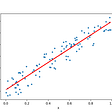# How Do Taylor Series Work?

## Discussing what to know about the Taylor Series

The Taylor Series is perhaps one of the most interesting topics that is covered in a calculus class. Certainly for me, learning about the Taylor Series was a daunting task full of twists and turns. However, the process was extremely rewarding, and the knowledge gained proved to be game changing on my outlook of calculus in general. In this article, I hope to articulate some of the concepts and ideas that I gathered about the Taylor Series.

The idea surrounding the Taylor Series is mostly attributed to Brook Taylor, who conducted worked on the concept in the early 18th century. The series itself is an infinite series of derivatives at a point that models a function inside a given interval, the interval of convergence. As the highest power on a given term, or the order of the series, rises, the model better approximates the function as a whole. The Taylor Series does not approximate the function well outside of the interval of convergence.

# Constructing the Series

A Taylor Series can be constructed at any x-coordinate, but there is a special name for a Taylor Series that is constructed at x = 0: the Mclaurin Series. We’ll start with this, and then generalize it to all Taylor Series. The formula of the Mclaurin Series of a function f with derivatives of all orders is

As you may notice, the series consists of terms that are increasing in the degree of their derivatives. For example, the second term contains the first derivative of the function, the third term contains the second derivative of the function, the fourth term contains the third derivative of the function etc. Of course, the first term of the series contains no derivative.

Imagine for a moment that we cut off everything but the first two terms. Does this look familiar? It is actually in fact the equation for the tangent line of a function f at x = 0. The f(0) part takes care of the initial point, while the f’(0) part takes care of the instantaneous rate of change. Add on the third term, and we take care of the instantaneous concavity, or the rate of change of the rate of change. With each additional term, another “degree of the rate of change” is covered by the series, better modeling the function in the process. Refer to some commonly used Mclaurin Series at the link below.

However, what if we want to model a function at a point that is not at x = 0, but rather at x = a? The formula then becomes the following.

The derivatives of all orders are now at the point x = a to account for this change in starting point. You’ll also notice that each of the “x” terms are now “shifted over” by a factor of a. Now, let’s look at some examples.

Suppose we have to find the third order Taylor Series for f(x) = x³ about the point x = 1. We begin by finding each of f(1), f’(1), f’’(1), and f’’’(1).

f(1) = 1

f’(1) = 3(1)² = 3

f’’(1) = 6(1) = 6

f’’’(1) = 6

Now, simply plug these values into our equation for a Taylor Series, we get

1 + 3(x-1) + (6/2!)(x-1)² + (6/3!)(x-1)³

= 1 + 3(x-1) + 3(x-1)² + (x-1)³

There are a few shortcuts that can be taken, because it may not always be pleasant to calculate multiple instantaneous derivatives. In fact, we can determine the Taylor/Mclaurin Series of a function in just a few seconds if it resembles one of the commonly used series.

Let’s say we wanted to find the Mclaurin Series for the function above. Just by looking at our f(x), it would be tedious to calculate the first, second, third derivatives. However, we recognize a familiar Taylor Series within f(x).

By recognizing the familiar function within our given function, we can manipulate the Taylor Series that we know to fit the Taylor Series that we want. If applicable, this method is most definitely the way to go as it saves precious time and energy.

# The Ratio Test

As mentioned before, a given Taylor Series may not converge for all real numbers. So, how do we find out for which values these types of series converge? The key is to use a test called The Ratio Test, which is found below

where the Taylor Series converges if C < 1 and diverges if C >1. If C = 1, then the ratio test is deemed inconclusive and further testing needs to be completed. The idea behind the ratio test falls back to the topic of geometric series. Geometric series are said to converge when the absolute value of the common ratio between the terms is less than one. If we look back at the ratio test, we can see that the same idea is being applied. Since the eventual behavior of the ratio is of importance here, the limit of the ratio is taken and convergence rules of geometric series can be applied because the ratio test is “as good as geometric.” Let’s dive into an example with the Mclaurin Series of the function ln(x + 1).

It is one of our “familiar” Mclaurin Series, so we know that it can be represented as the infinite series.

From here on out, all that needs to be done is to plug in the necessary terms into the ratio test formula and simplify.

We have now essentially obtained our common ratio, and the next step is to set this common ratio to be less than 1. Doing so yields the interval -1 < x < 1. The last step that needs to be done is to check for convergence at the endpoints of the interval. If we plug in -1, we see that the series is the divergent harmonic series while if we plug in 1, the series is the convergent alternating harmonic series. Therefore we have our final interval of convergence.

# Wrapping Up

Learning about the Taylor Series was by far the highlight of my calculus class. It pushed me to refine my mechanics and also push my conceptual thinking to a level that I had not previously reached. The fact that we can write functions that approximate polynomials infinitely well truly opened my mind up to the ever-expanding branches of mathematics. If there’s one subject within calculus that you should pay the most attention to, it should be the Taylor Series.

--

--

--

## More from Albert Ming

18 || High School Senior || Stats & Math

Love podcasts or audiobooks? Learn on the go with our new app.

## Number System’s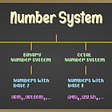## RL — Actor-Critic using Kronecker-Factored Trust Region (ACKTR) Explained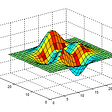## Geodesic Regression## An Intuitive Explanation of Benford’s Law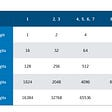## How to win 4d## Emanuel Lasker: Mathematician who is the Longest Reigning World Chess Champion## Quantum Algo: Shor Algorithm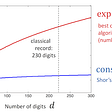## Albert Ming

18 || High School Senior || Stats & Math

## Pigeonhole Principle and Functions## Simple Pigeonhole principle, but interesting result — Journey in Combinatorics## Matrix & Matrix Operations | Numerical Linear Algebra | Part 1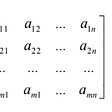## Regressions Explained